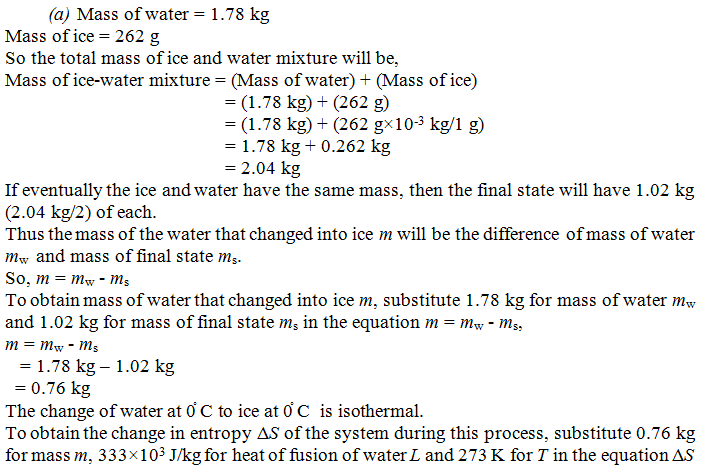Click to Chat

1800-1023-196

+91-120-4616500

CART 0

• 0

MY CART (5)

Use Coupon: CART20 and get 20% off on all online Study Material

ITEM
DETAILS
MRP
DISCOUNT
FINAL PRICE
Total Price: Rs.

There are no items in this cart.
Continue Shopping
`        A mixture of 1.78 kg of water and 262 g of ice at 0ºC is, in a reversible process, brought    to a final equilibrium state where The water/ice ratio, by mass, is 1: 1 at 0ºC. (a) Calculate the entropy change of the system during this process. (b) The system is then returned to the first equilibrium state, but in an irreversible way (by using a Bunsen burner, for instance). Calculate the entropy change of the system during this process. (c) Show that your answer is consistent with the second law of thermodynamics.`
4 years ago

```= -mL/T,ΔS = -mL/T = -(0.76 kg) (333×103 J/kg )/(273 K) = -927 J/KFrom the above observation we conclude that, the change in entropy ΔS of the system during this process will be -927 J/K.(b) Now the system is returned to the first equilibrium state, but in an irreversible way. Thus the change in entropy ΔS of the system during this process is equal to the negative of previous case.So, ΔS = -(- 927 J/K) = 927 J/KFrom the above observation we conclude that, the change in entropy ΔS of the system would be 927 J/K.(c) In accordance to second law of thermodynamics, entropy change ΔS is always  zero.The total change in entropy will be,ΔS = (-927 J/K) + (927 J/K) = 0From the above observation we conclude that, our answer is consistent with the second law of thermodynamics.
```
4 years ago
Think You Can Provide A Better Answer ?

## Other Related Questions on Thermal Physics

View all Questions »### Course Features

• 101 Video Lectures
• Revision Notes
• Previous Year Papers
• Mind Map
• Study Planner
• NCERT Solutions
• Discussion Forum
• Test paper with Video Solution### Course Features

• 110 Video Lectures
• Revision Notes
• Test paper with Video Solution
• Mind Map
• Study Planner
• NCERT Solutions
• Discussion Forum
• Previous Year Exam Questions